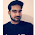## Saturday, June 9, 2018

Test your Mathematical skills as well as Logical reasoning skills with the Math Logic Question. In this Interesting Math Logic Puzzle, you are given some number equations. In these number equations, numbers on the left side of equations relate to the number on the right side of the equation. Can you find this logical relationship between these number equations and then solve the last equation to find the value of missing number which will replace the question mark?Can you solve this math logic question?

Answer of this "Math Logic Question and Answer for Students", is hidden as of now to avoid any spoiler. Do post your answers in the comments box. Also, you can post your request to view the answer in the comment section. Please do give your best try to solve this brain teaser.

1.1.Your answer differs from our answer for this math logic question. It will be great if you can write the logic used to solve this puzzle?

2.I don't know tell me the right ans

1.Thanks for asking the answer for this math logic puzzle. I have now posted the logical reasoning used to solve this puzzle along with the answer.
Please do let me know if something is not clear regarding the logic or answer for this puzzle?

3.A comment doesn't cost a thing. Please drop a comment below to boost the author's morale.